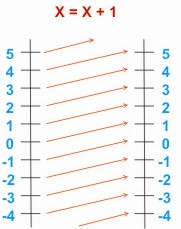Question: What do you mean by Mapping ? Answer: A mapping is another name for a function. It comes from the geographical idea of making a map in which for each point on the ground there is a unique point on the map. This idea of transforming one set of points to another is used in mathematics, and transformations such as reflections, rotations, translations, enlargements, etc. are referred to as mappings. Similarly, functions such as x __> x + 1. where x is a real number, are sometimes called mappings. x __> x + 1 maps the number line onto itself, each number having 1 added on. It can be using a mapping diagram as shown :